RS Aggarwal Class 6 Solutions Chapter 14- Constructions Ex 14A(14.1)

RS Aggarwal Class 6 Chapter 14- Constructions Ex 14A(14.1) Solutions Free PDF

Question 1:

Using a pair of compasses construct of the following angle:

(i) 60o

(ii) 120o

(iii) 90o

Solution: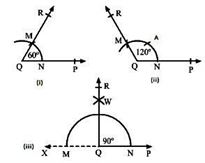(i)

Steps for construction:

1. Draw a ray QP.

2. With Q as the centre and any convenient radius, draw an arc cutting QP at N.

3. With N as the centre and the same radius as before, draw another arc to cut the previous arc at M.

4. Draw QM and produce it to R.

$\angle$PQR is the required angle of 60o.

(ii)

Steps for construction:

1. Draw a ray QP.

2. With Q as the centre and any convenient radius, draw an arc cutting QP at N.

3. With N as the centre and the same radius, cut the arc at A. Again, with A as the centre and the same radius, cut the arc at M.

4. Draw QM and produce it to R.

$\angle$PQR is the required angle of 120o.

(iii)

Steps for construction:

1. Draw a line PX.

2. Take a point Q on AC. With Q as the centre and any convenient radius, draw an arc cutting AX at M and N.

3. With N as the centre and radius more than half on MN, draw an arc.

4. With M as the centre and the same radius as before, draw another arc to cut the previous arc at W.

5. Draw QW and produce it to R.

$\angle$PQR is required angle of 90o.

Question 2:

Draw an angle of 60o, using a pair of compasses. Bisect it to make an angle of 30o.

Solution:

Constructions steps: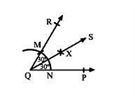1. Draw a ray QP.

2. With Q as the centre and any convenient radius, draw an arc cutting QP at N.

3. With N as the centre and radius same as before, draw another arc to cut the previous arc at M.

4. Draw QM and produce it to R.

$\angle$PQR is required angle of 60o

5. With M as the centre and radius more than half of MN, draw an arc.

6. With N as the centre and radius same as in step (5), draw another arc, cutting the previously drawn arc at point X.

7. Draw QX and produce it to point S.

Ray QS is the bisector of $\angle$PQR.

Question 3:

Draw an angle of 45o, using a pair of compasses.

Solution:

Construction steps: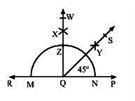1. Draw a line PR.

2. Take a point Q on PR. With Q as the centre and any convenient radius, draw an arc cutting AC at M and N.

3. With N as the centre and radius more than half of MN, draw an arc.

4. With M as the centre and the same radius as before, draw another arc to cut the previous arc at X.

5. Draw QX, meeting the arc at Z produce it to W.

6. With Z as the centre and radius more than half of ZN, draw an arc.

7. With N as the centre and the same radius as in step (6), draw another arc, cutting the previously drawn arc at a point Y.

8. Draw QY and produce it to point S.

$\angle$PQS is the required angle of 45o.

Question 4:

Use a pair of compasses and construct the following angles:

(i) 150o

(ii) 15o

(iii) 135o

(iv) $22\frac{1}{2}^{\circ}$

(v) 105o

(vi) 75o

(vii) $67\frac{1}{2}^{\circ}$

(viii) 45o

Solution:

(i)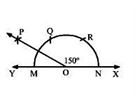Steps for construction:

1. Draw a line XY and take a point O.

2. With O as the centre and any suitable radius, draw an arc cutting XY at M and N.

3. With N as the centre and the same radius, draw an arc cutting MN at R.

4. With R as the centre and the same radius as before, draw another arc cutting MN at Q.

5. With Q as the centre and radius less than MQ draw an arc.

6. With M as the centre and the same radius draw another arc cutting the previously drawn arc at P.

7. Join PO.

Therefore, $\angle$XOP = 150o

(ii)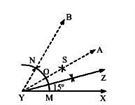Steps for construction:

1. Draw a ray XY.

2. With X as the centre and any convenient radius, draw an arc cutting XY at M.

3. With M as the centre and the same radius, draw an arc cutting the previously drawn arc at N.

4. Draw YN and produce it to B.

5. Draw the bisector AY of $\angle$XYB.

6. Again, draw the bisector YZ of $\angle$XYA.

Therefore, $\angle$XYZ = 15o

(iii)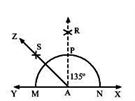Steps for construction:

1. Draw a line XY and take a point A.

2. With A as the centre and any convenient radius, draw an arc cutting XY at M and N.

3. With N as the centre and the same radius, draw an arc.

4. With M as the centre and the same radius as before, draw another arc cutting the previously drawn arc at R.

5. Draw RA.

6. Draw the bisector ZA of $\angle$YAR.

Therefore, $\angle$XAZ = 135o

(iv)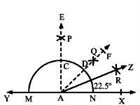Steps for construction:

1. Draw a line XY.

2. Take a point A on XY. With A as the centre and any convenient radius, draw an arc cutting XY at M and N.

3. With N as the centre and radius more than half of MN, draw an arc.

4. With M as the centre and the same radius as before, draw another arc to cut the previous arc at P.

5. Draw PA meeting the arc at C. Produce it to E.

6. With C as the centre and radius more than half of CN, draw an arc.

7. With N as the centre and the same radius as in step (6), draw another arc cutting the previously drawn arc at a point Q.

8. Draw AQ and produce it to point F.

9. Draw the bisector ZA of $\angle$XAF.

Therefore, $\angle$XAZ = 22.5o

(v)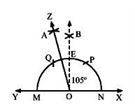Steps for construction:

1. Draw a line XY.

2. Take a point O on XY. With O as the centre and any convenient radius, draw an arc cutting XY at M and N. Draw arcs with the same radius cutting MN at P and Q.

3. With N as the centre and the radius more than half of MN, draw an arc.

4. With M as the centre and the same radius as before, draw another arc to cut the previous arc at B.

5. Draw BO meeting the arc at E.

6. With Q as the centre and radius more than half of PE, draw an arc.

7. With E as the centre and the same radius as in step (6), draw another arc cutting the previously drawn arc at a point A.

8. Draw AO and produce it to point Z.

Therefore, $\angle$XOZ = 105o

(vi)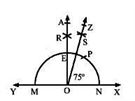Steps for construction:

1. Draw a line XY.

2. Take a point O on XY. With O as the centre and any convenient radius, draw an arc cutting XY at M and N. Draw arcs with the same radius cutting MN at P.

3. With N as the centre and radius more than half of MN, draw an arc.

4. With M as the centre and the same radius as before, draw another arc to cut the previous arc at R.

5. Draw RO meeting the arc at E. Produce it to A.

6. With P as the centre and radius more than half of PE, draw an arc.

7. With E as the centre and the same radius as in step (6), draw another arc cutting the previously drawn arc at the point S.

8. Draw OS and produce it to point Z.

Therefore, $\angle$XOZ = 75o

(vii)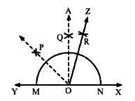Steps for construction:

1. Draw a line XY and take a point O.

2. With O as the centre and any convenient radius, draw an arc cutting XY at M and N.

3. With N as the centre and the same radius, draw an arc.

4. With M as the centre and the same radius as before, draw another arc cutting the previously drawn arc at Q.

5. Draw QO.

6. Draw PO bisector of $\angle$YOA.

7. Draw ZO bisector of $\angle$POX.

Therefore, $\angle$XXAZ = 67.5o

(viii)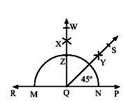Steps for construction:

1. Draw a line PR.

2. Take a point Q on PR. With Q as the centre and any convenient radius, draw an arc cutting AC at M and N.

3. With N as the centre and radius more than half of MN, draw an arc.

4. With M as the centre and the same radius as before, draw another arc to cut the previous arc at X.

5. Draw QX, meeting the arc at Z. Produce it to W.

6. With Z as the centre and radius more than half of ZN, draw an arc.

7. With N as the centre and the same radius as in step (6), draw another arc cutting the previously drawn arc to a point Y.

8. Draw QY and produce it to point S.

Question 5:

Draw a rectangle whose two adjacent sides are 5 cm and 3.5 cm. Make use of a pair of compasses and a ruler only.

Solution:

Construction steps: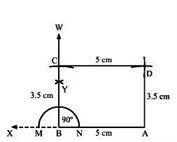1. Draw a ray AX.

2. With A as the center, cut the ray AX at B such that AB is equal to 5 cm.

3. With B as the center and any convenient radius, draw an arc cutting AX at M and N.

4. With N as the center and radius more than half of MN, draw an arc.

5. With M as the center and the same radius as before, draw another arc to cut the previous arc at Y.

6. Draw BY and produce it to W.

7. With B as the center and a radius of 3.5 cm, cut ray BW at point C.

8. With C as the center and a radius of 5 cm, draw an arc on the right side of BC.

9. With A as the center and a radius of 3.5 cm, draw an arc cutting the previous arc at D.

ABCD is the required rectangle.

Question 6:

Draw a square, each of whose side is 5 cm. Use a pair of compasses and a ruler in your construction.

Solution:

Construction steps: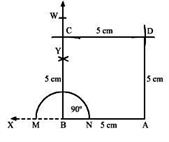1. Draw a ray AX.

2. With A as centre cut the ray AX at B such that AB = 5 cm.

3. With B as centre and any convenient radius, draw an arc cutting AX at M and N.

4. With N as centre and radius more than half of MN draw an arc.

5. With M as centre and the same radius as before, draw another arc to cut the previous arc at Y.

6. Join BY and produced it to W.

7. With B as centre and radius 5 cm cut ray BW at point C.

8. With C as centre and radius 5 cm draw an arc on right side of BC.

9. With A as centre and radius 5 cm draw an arc cutting the previous arc at D.